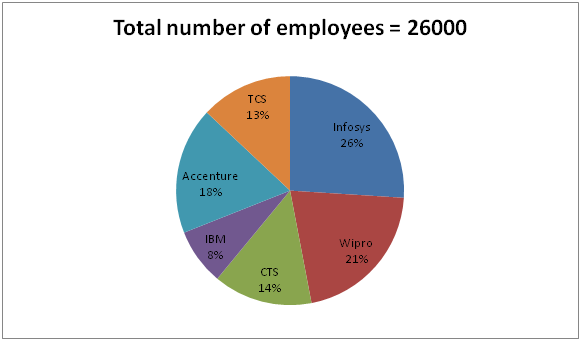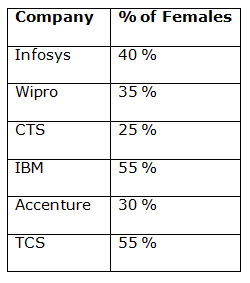# SBI PO Prelims Quantitative Aptitude Questions 2019 – Day 04

SBI PO 2019 Notification will be expected soon. It is one of the most expected recruitment among the banking aspirants. Every year the exam pattern for SBI PO has been changing. Depends upon the changing of exam pattern the questions are quite harder compare to the previous year. So the questions are in high level than the candidate’s assumption.

As per the latest trend, our IBPS Guide is providing the updated New Exam Pattern Quantitative Aptitude questions for SBI PO 2019 Day 4. Our Skilled experts were mounting the questions based on the aspirant’s needs. So candidates shall start your preparation and practice on daily basis with our SBI PO pattern quantitative aptitude questions 2019 day 4. Start your effective preparation from the right beginning to get success in upcoming SBI PO 2019.

[WpProQuiz 5010]

### Click Here for SBI PO Pre 2019 High-Quality Mocks Exactly on SBI Standard

1) In a class of ____ students, number of girls in the class is 6 more than the number of boys in the class. The average weight of the all the students in the class is 42.25 Kg. Average weight of the boys is ____ Kg and average weight of the girls is 40 Kg. Which of the following option satisfies the given condition?

a) 50, 42

b) 40, 45

c) 60, 45

d) 46, 42

e) None of these

2) The current of a stream runs with the speed of _______ Km/h. A motor boat travels 120 Km downstream and 108 Km upstream in 15 hours. Speed of the motorboat in still water is _______.

Which of the following options satisfy the given condition?

I. 4, 16

II. 5, 15

III. 2, 8

a) Only I

b) Only II

c) Only III

d) Both I and II

e) Both II and III

3) Mohana invested Rs._____ on simple interest at 8% per annum for 5 years on scheme X. She invested Rs.75000 on compound interest at 12% per annum for two years on scheme Y. Difference between the total amount got by him from scheme X and scheme Y is Rs._____.

Which of the following options satisfy the given condition?

I. 40000, 38080

II. 55000, 17080

III. 60000, 15000

a) Both I and II

b) Both II and III

c) Both I and III

d) All I, II and III

e) Only III

4) If the numerator of a fraction is increased by 250% and denominator of the fraction is increased by ________% the fraction becomes 2/3. The original fraction is ____________. Which of the following options satisfy the given condition?

a) 300, 5/6

b) 150, 4/5

c) 100, 3/4

d) 200, 4/7

e) None of these

5) Four friends Anita, Binita, Krishna and Mrinal start running around a circular field and complete one round in 4, ____, 10 and 15 seconds respectively. After ______ minutes they will meet again at the starting point for the first time after they start.

Which of the following options satisfy the given condition?

a) 6, 3

b) 8, 2

c) 5, 2

d) 2, 3

e) None of these

Directions (6 – 10): Study the following information carefully and answer the given questions.

The following pie chart shows total number of employees in different MNC companies and the table shows the percentage of females in the companies.6) Total number of female employees in Infosys and IBM is approximately what percentage more/less than the total male employees in Accenture and Wipro?

a) 58 % more

b) 44 % less

c) 32 % more

d) 32 % less

e) 58 % less

7) Find the ratio between the total number of male employees in CTS to the total number of female employees in Accenture?

a) 35 : 18

b) 27 : 14

c) 32 : 17

d) 28 : 19

e) None of these

8) Find the average number of male employees in TCS, CTS and IBM?

a) 1875

b) 1687

c) 1729

d) 1574

e) None of these

9) Which company has highest number of female employees?

a) TCS

b) Accenture

c) IBM

d) Infosys

e) None of these

10) Total number of employees of Infosys is approximately what percentage of total number of employees of Accenture?

a) 128 %

b) 144 %

c) 136 %

d) 112 %

e)125 %

Let, the number of boys = n

=> The number of girls = n + 6

Option (a)

Total number of students in the class = 50

n + n + 6 = 50

=> 2n = 50 – 6

=> 2n = 44

=> n = 22

Boys = 22

Girls = 22 + 6 = 28

(22 x 42) + (28 x 40) = 924 + 1120 = 2044 [50 x 42.25 =2112.5]

This does not satisfy the given condition.

Option (b)

Total number of students in the class = 40

n + n + 6 = 40

=> 2n = 40 – 6

=> 2n = 34

=> n = 17

Boys = 17

Girls = 17 + 6 = 23

(17 x 45) + (23 x 40) = 765 + 920 = 1685 ≠ [40 x 42.25 = 1690]

This does not satisfy the given condition.

Option (c)

Total number of students in the class = 60

n + n + 6 = 60

=> 2n = 60 – 6

=> 2n = 54

=> n = 27

Boys = 27

Girls = 27 + 6 = 33

(27 x 45) + (33 x 40) = 1215 + 1320 = 2535 = [60 x 42.25 = 2535]

This satisfies the given condition.

Option (d)

Total number of students in the class = 46

n + n + 6 = 46

=> 2n = 46 – 6

=> 2n = 40

=> n = 20

Boys = 20

Girls = 20 + 6 = 26

(20 x 42) + (26 x 40) = 840 + 1040 = 1880 ≠ [46 x 42.25 = 1943.5]

This does not satisfy the given condition.

I.

120/(16 + 4) + 108/(16 – 4)

= 120/20 + 108/12

= 6 + 9

= 15 hours

This satisfies the given condition.

II.

120/(15 + 5) + 108/(15 – 5)

= 120/20 + 108/10

= 6 + 10.8

= 16.8 hours ≠ 15 hours

This does not satisfy the given condition.

III.

120/(8 + 2) + 108/(8 – 2)

= 120/10 + 108/6

= 12 + 18

= 30 hours ≠ 15 hours

This does not satisfy the given condition.

We know that

Amount on CI = P x (1 + r/100)t

= 75000 x (1 + 12/100)2

= 75000 x (112/100) x (112/100)

= Rs. 94080

I.

We know that

Amount on SI = [(P x r x t)/100] + P

= [(40000 x 8 x 5)/100] + 40000

= 16000 + 40000

= Rs. 56000

Difference = 94080 – 56000 = Rs. 38080

This satisfies the given condition.

II.

We know that

Amount on SI = [(P x r x t)/100] + P

= [(55000 x 8 x 5)/100] + 55000

= 22000 + 55000

= Rs. 77000

Difference = 94080 – 77000 = Rs.17080

This satisfies the given condition.

III.

We know that

Amount on SI = [(P x r x t)/100] + P

= [(60000 x 8 x 5)/100] + 60000

= 24000 + 60000

= Rs.84000

Difference = 94080 – 84000 = Rs.10080 ≠ 15000

This does not satisfy the given condition.

Option (a)

(5 x 350/100)/(6 x 400/100)

= 35/48 ≠ 2/3

This does not satisfy the given condition.

Option (b)

(4 x 350/100)/(5 x 250/100)

= 28/25 ≠ 2/3

This does not satisfy the given condition.

Option (c)

(3 x 350/100)/(4 x 200/100)

= 21/16 ≠ 2/3

This does not satisfy the given condition.

Option (d)

(4x 350/100)/(7 x 300/100)

= 2/3

This satisfies the given condition.

Option (a)

Time after they will meet again = LCM of 4, 6, 10, 15 = 60 seconds = 1 minute

This does not satisfy the given condition.

Option (b)

Time after they will meet again = LCM of 4, 8, 10, 15 = 120 seconds = 2 minutes

This satisfies the given condition.

Option (c)

Time after they will meet again = LCM of 4, 5, 10, 15 = 60 seconds = 1 minute

This does not satisfy the given condition.

Option (d)

Time after they will meet again = LCM of 4, 2, 10, 15 = 60 seconds = 1 minute

This does not satisfy the given condition.

Direction (6-10) :

Total number of female employees in Infosys

= > 26000*(26/100)*(40/100) = 2704

Total number of female employees in IBM

= > 26000*(8/100)*(55/100) = 1144

Total number of female employees in Infosys and IBM = 2704 + 1144 = 3848

Total male employees in Accenture

= > 26000*(18/100)*(70/100) = 3276

Total male employees in Wipro

= > 26000*(21/100)*(65/100) = 3549

Total male employees in Accenture and Wipro = 3276 + 3549 = 6825

Required %

= > [(6825 – 3848)/6825]*100 = 297700/6825= 43.61 % = 44 % less

Total number of male employees in CTS

= > 26000*(14/100)*(75/100) = 2730

Total number of female employees in Accenture

= > 26000*(18/100)*(30/100) = 1404

Required ratio = 2730: 1404 = 35: 18

Total number of male employees of TCS, CTS and IBM

= > 26000*(13/100)*(45/100) + 26000*(14/100)*(75/100) + 26000*(8/100)*(45/100)

= > 1521 + 2730 + 936

= > 5187

Average number of male employees = 5187/3 = 1729

Total number of female employees of Infosys

= > 26000*(26/100)*(40/100) = 2704

Total number of female employees of Wipro

= > 26000*(21/100)*(35/100) = 1911

Total number of female employees of CTS

= > 26000*(14/100)*(25/100) = 910

Total number of female employees of IBM

= > 26000*(8/100)*(55/100) = 1144

Total number of female employees of Accenture

= > 26000*(18/100)*(30/100) = 1404

Total number of female employees of TCS

= > 26000*(13/100)*(55/100) = 1859

Infosys has highest number of female employees.

Total number of employees of Infosys

= > 26000*(26/100) = 6760

Total number of employees of Accenture

= > 26000*(18/100) = 4680

Required % = (6760/4680)*100 = 144.44 % = 144 %

### Click Here for SBI PO Pre 2019 High-Quality Mocks Exactly on SBI Standard